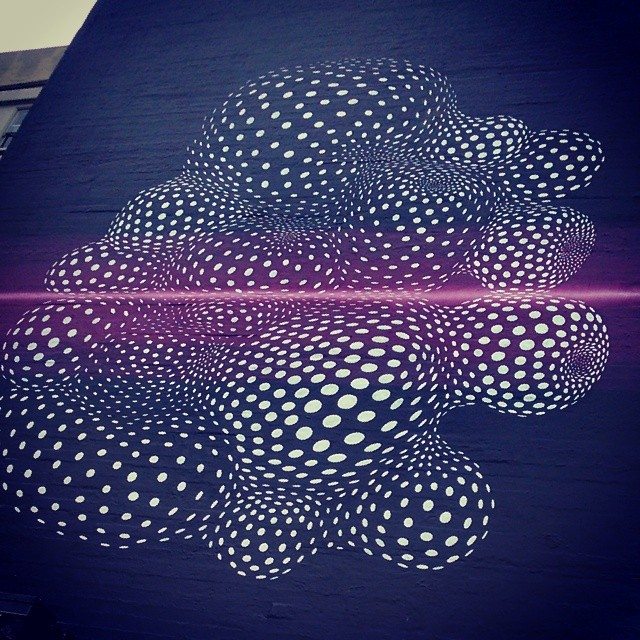## Technology

Notes

Randoms

July 2014It's more difficult than you'd imagine, plucking random digits from a computer. 'Puters are rational beasts that only follow very specific instructions.

A random number is, by its very definition, something that can't be anticipated by an algorithmic formula. Yet, computers are called upon all the time to produce random output. Picking songs from a playlist at random, for example.

"In a sense, there is no such thing as a random number; for example, is 2 a random number? Rather, we speak of a sequence of independent random numbers with a specified distribution," writes the godfather of computer science, Donald Knuth, in the Art of Computer Programming, Volume 2: Seminumerical Algorithms, 3rd Edition.

"Each number was obtained merely by chance, having nothing to do with other numbers of the sequence," he notes in the chapter on randomness. "Each number has a specified probability of falling in any given range of values."

Weirdly, true randomness is not always the best random experience, for the human anyway. In true randomness, there is both oversampling and undersampling, as Knuth pointed out. This is why Apple several years back developed a pseudo-random option for iTunes random play, so the music player would cast its attentions more evenly across all the choices when in shuffle mode. True random is more monotonous than pseudo random.

How can you tell if a series of digits are truly random? The answer can be quite philosophically – and mathematically -- complex.

Here's one trick: If a sequence is truly random, then no algorithm created to replicate that sequence could be any shorter in length than the sequence itself.

A sequence can be considered random if the only way it could be recreated would involve listing every exact number, one by one, in that sequence. This here is called the Kolmogorov Complexity.

```

```